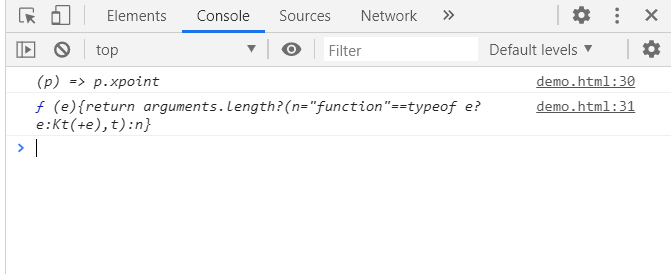# D3.js line.x() method

• Last Updated : 17 Aug, 2020

The d3.line.x() method sets or gets the x accessor point of the line. If x is provided, it must be a number or a function that returns a number.

Syntax:

`d3.line.x(x-point);`

Parameters:

• x-point: This method takes an x-point that can be set from the points array.

Return Value: This method returns the x accessor point of the line.

Example 1: Setting the x-point using this method. For y-points here we have used line.y() function.

 ```<``html``>``<``meta` `charset``=``"utf-8"``>``<``head``>``  ``<``title``>Line in D3.js````<``script` `src``=``"https://cdnjs.cloudflare.com/ajax/libs/d3/4.2.2/d3.min.js"``>```` ` `<``body``>``    ``<``h1` `style="text-align: center;``               ``color: green;">``         ``GeeksforGeeks``   ````  ``<``center``>``    ``<``svg` `id``=``"gfg"` `width``=``"200"` `height``=``"200"``>``  ``````  ``<``script``>``var points = [``  ``{xpoint: 25,  ypoint: 150},``  ``{xpoint: 75,  ypoint: 85},``  ``{xpoint: 100, ypoint: 115},``  ``{xpoint: 175, ypoint: 25}];`` ` `var Gen = d3.line()``  ``// Setting the x-point``  ``.x((p) => p.xpoint)``  ``.y((p) => p.ypoint);`` ` `d3.select("#gfg")``  ``.append("path")``  ``.attr("d", Gen(points))``  ``.attr("fill", "none")``  ``.attr("stroke", "green");```````

Output:Example 2: getting the function for x points.

 ```<``html``>``<``meta` `charset``=``"utf-8"``>``<``head``>``  ``<``title``>Line in D3.js````<``script` `src``=``"https://cdnjs.cloudflare.com/ajax/libs/d3/4.2.2/d3.min.js"``>```` ` `<``body``>``    ``<``h1` `style``=``"text-align: center; color: green;"``>``       ``GeeksforGeeks``    ````  ``<``center``>``    ``<``svg` `id``=``"gfg"` `width``=``"200"` `height``=``"200"``>````  ``<``script``>``var points = [``  ``{xpoint: 25,  ypoint: 150},``  ``{xpoint: 75,  ypoint: 85},``  ``{xpoint: 100, ypoint: 115},``  ``{xpoint: 175, ypoint: 25}];`` ` `var Gen = d3.line()``  ``.x((p) => p.xpoint)``  ``.y((p) => p.ypoint);`` ` `d3.select("#gfg")``  ``.append("path")``  ``.attr("d", Gen(points))``  ``.attr("fill", "none")``  ``.attr("stroke", "green");``console.log(Gen.x());``console.log(Gen.x)```````

Output:My Personal Notes arrow_drop_up